telescopeѲptics.net          ▪▪▪▪                                             CONTENTS

10.2.3. Full-aperture meniscus corrector

PAGE HIGHLIGHTS
Maksutov corrector   • Coma   • Astigmatism

Around 1941 Anton Bowers and Dmitry Maksutov (according to Rutten and Venrooij, also Gabor and Penning) separately arrived at a similar idea on how to minimize aberrations of a spherical mirror. They proposed placing concentric, or near-concentric meniscus lens at some distance in front of the mirror. If positioned properly, and with needed optical properties, such meniscus could eliminate off-axis aberrations of the mirror, and greatly reduce its spherical aberration.

Bowers

For the concentric meniscus form, the distance to a mirror is determined from the concentric configuration for all three surfaces needed for the cancellation of off-axis aberrations (FIG. 181). Obviously, meniscus radii are related to the center thickness t as t=R1-R2, for the front and rear radius, respectively, with both radii being nominally negative. Since the corrector is a weak negative lens, the resulting combined focus after reflection from the mirror is formed somewhat farther away from the mirror focus. However, the effective nominal focal length is somewhat smaller than mirror's focal length, due to the wavefront having larger diameter at the primary than at the corrector (aperture stop).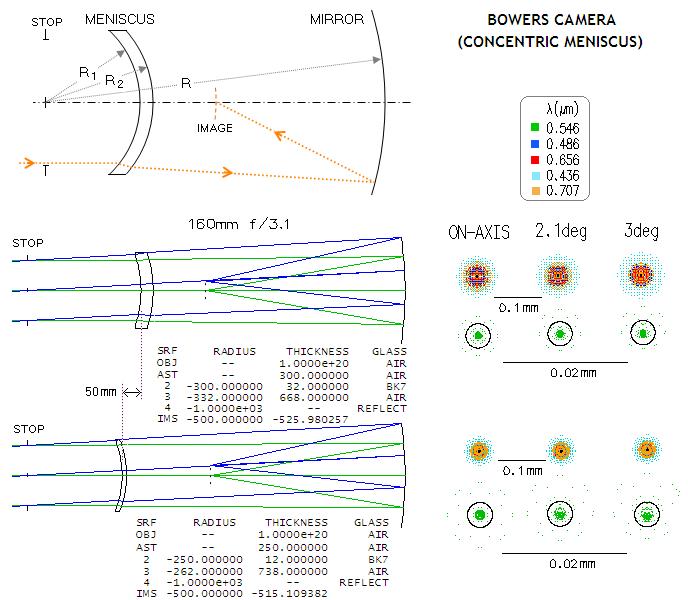FIGURE 181
: Concentric meniscus correcting monochromatic aberrations of spherical mirror. All three optical surfaces and the Petzval image surface have a common center of curvature, coinciding with the center of the aperture stop (top). As a result, from the position of the aperture stop, the system has no unique axis, which makes it free from off-axis aberrations. Meniscus thickness is given by t=R
1-R2, with both lens radii numerically negative (concave to the left). Obviously, the stop to mirror separation is identical to that with the Schmidt camera (assuming stop at the corrector). There is no unique axial location for the meniscus, since at any location it can be made so that it generates spherical aberration needed to offset that of the mirror. However, choice of location does affect chromatic correction and the level of higher order spherical. Ray trace above illustrates the difference in corrector properties and correction level resulting from a relatively small change in meniscus location relative to the stop and the mirror in a 160mm ƒ/3 camera. Meniscus closer to the mirror has to be thicker, because its radii are weaker and the increase in thickness is necessary to generate more of a differential in the spherical aberration generated at its two surfaces. The thicker corrector has about 1/3 better correction in the optimized wavelength, due to less higher-order spherical generated at its more weakly curved surfaces, but has noticeably larger longitudinal chromatism, which is already unacceptable with the thinner meniscus (note the different scales for polychromatic and monochromatic blurs). Correction in the optimized wavelength is near perfect over the 3-degree field radius. The only drawback of the concentric meniscus is its large longitudinal chromatism, making it unsuitable for fast cameras.

Concentric meniscus' second principal plane coincides with its center of curvature. Consequently, while it is a thick lens, it can be considered to be a thin lens of the focal length ƒ~ƒL-R1, with ƒL being the focal length of the concentric meniscus derived from the thick lens f.l. relation, ƒL=nR1R2/(n-1)t, and R1 the front surface radius of curvature), placed at the center of curvature of its two surfaces. With R1<<ƒL, the aberration relations for a thin lens of the focal length ƒ~ƒL can be applied with ~1% error (negative) in the value of the spherical aberration coefficient. By substituting p=-1 and q in terms of ƒL and R1 into Eq. 49, the aberration coefficient for 3rd order spherical aberration of the meniscus is closely approximated by: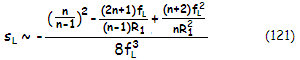n being the lens refractive index. With R1«ƒL, the first two terms in the numerator are much smaller than the third, and it can be simplified to:Since R1 is, for an ~ƒ/3 mirror, on the order of 50 times smaller than ƒL, this approximation will likely give a  6-7% larger value than the actual. However, since the meniscus also generates higher order spherical of the same sign, and roughly similar in magnitude, the approximation gives a fairly good assessment of the total aberration load of the meniscus.

It should be emphasized though, that this is only valid if meniscus does behave like thin lens. This is not entirely so, and it may not be negligible. While it does fulfill the thin lens condition of the focal length being much larger than the diameter, its strongly curved surfaces partly defy the purpose of this condition, which is that the ray height on the two opposite sides of the lens are nearly identical, and that the image created by the first surface is at nearly equal distance from both, first and second surface. With the marginal ray being appreciably higher at the second surface (in light of spherical aberration changing with the 4th power of the height), and the second surface being appreciably farther from the (virtual) image formed by the first surface than the first surface itself, the aberration contribution of the second surface - which is undercorrection - is appreciably higher than what the thin lens equation imply. Since overcorrection generated by the first surface is dominant, this means that overcorrection generated by the meniscus is somewhat lower - approximately, up to 4-5% with an ~f/3 mirror - than what the thin lens treatment implies.

In order to cancel spherical aberration of the mirror, the meniscus needs to generate identical amount of the aberration, but of the opposite sign (over-corrected). With the mirror aberration coefficient for primary spherical aberration sP=1/4R3 (for spherical mirror), R being the mirror radius of curvature, the aberration coefficient for the combination is given by a sum of the two, and the P-V wavefront error of 3rd order spherical aberration at the best focus, after substitution for the meniscus' focal length ƒL=-nR1R2/(n-1)t, and multiplying with (D/2)4 divided by 4 for the best focus error, is closely approximated by: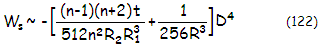with R2 being the rear meniscus surface radius of curvature, t the corrector center thickness and D the aperture diameter. Obviously, zero system aberration requires zero sum in the brackets which, in turn, for given index of refraction n, requires appropriate values for the three radii and meniscus thickness. As mentioned, this approximated corrector contribution is up to 6-7% larger than the actual, and that of the primary - due to the larger effect of ray height increase at the primary on one, and smaller opposing effect of ray divergence caused by the corrector (effectively placing the object for the primary approximately at the distance equaling corrector's focal length), that are unaccounted for - is roughly as much smaller than the actual, making this approximate expression err by 10-15%. Including the higher order contribution of the meniscus (that of the mirror is near negligible in comparison), which is of the same sign as its lower order aberration, roughly cuts the error in half.

Since the focal length of the meniscus - which is a week negative lens - changes with the wavelength, so does the system focal length, ƒs=[1/(1-ƒm/ƒL]ƒm, resulting in longitudinal chromatism (ƒm is the mirror focal length, with all three focal lengths being numerically negative), given by LCs=ιtƒs2/R1R2n2 or, with the system focal length ƒs~R/2, closely approximated by: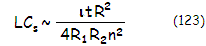with ι being the index differential vs. optimized wavelength. It can't be directly compared to the chromatism of the Schmidt camera, which does not generate longitudinal chromatism, but the defocus blur of the concentric meniscus is comparable to the spherical aberration blur (least circle of confusion) with the 0.866 neutral zone Schmidt with respect to the wavefront error generated. With the blur diameter for the concentric meniscus given by LCs/F, and for the Schmidt by Eq. 108.2, typical concentric meniscus corrector (t~D/10, R1=R/5 to R/4, and R1~0.94R2) has longitudinal chromatism greater by a factor of ~43(n-1)F/n2.

However, since the minimum spherochromatic error with the 0.707 neutral zone Schmidt corrector is more than twice smaller than with 0.866 neutral zone, the ratio is about 90(n-1)F/n2. With n=1.5, an ƒ/3 camera with concentric meniscus would have approximately 60 times greater wavefront error of chromatism in any non-optimized wavelength. Since chromatism of the Schmidt camera itself may become excessive at large relative apertures, it is much more of a problem for the concentric meniscus.

One solution to the excessive chromatism is to make concentric meniscus out of two different glasses cemented together.

Maksutov

Another solution, found by Maksutov, is to make the focal length invariant of the wavelength. In other words, while with the concentric meniscus rays falling onto the mirror appear as if coming from the focus formed by the meniscus, varying in its longitudinal placement (i.e. focal length) with the wavelength, with non-concentric Maksutov corrector they appear as if coming from the same focus point. This requires change in the meniscus radii/thickness relationship to: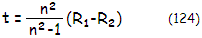The radii are no longer concentric, hence this achromatic, or Maksutov corrector is also called non-concentric.

For n~1.5, this makes the achromatic meniscus corrector thicker by a factor of 1.8. Also, for equal thickness/radii, the meniscus focal length is now longer by a factor of n/(n-1), given by ƒL=-n2R1R2/(n-1)2t. With the radii not being concentric anymore, low, but not quite negligible off-axis aberrations are reintroduced. However, they are easily dealt with by minor adjustments of the stop (corrector) location.

This meniscus modification practically eliminates longitudinal chromatism. Remaining spherochromatism - caused by the variation of spherical aberration with the wavelength - is minimized by bringing best foci of different wavelengths close together, while widening paraxial foci separation (FIG. 183, b and c). This requires slight correction of the rear radius, which is made slightly weaker than indicated by Eq. 124: R2=R1-[1-(1/n2)]t, which brings paraxial foci close together is replaced by  R2=R1-[1-(1/n2)]t/0.97, which brings together best foci of different wavelengths, usually corresponding to those of 0.8-0.9 zone rays (note that both R1 and R2 are numerically negative, while the thickness t is of positive sign).

More serious problem of the achromatized meniscus - also shared by the concentric version - is the amount of higher order spherical aberration. Due to the very strongly curved lens surfaces, higher-order spherical aberration becomes rather severe at large relative apertures. By reintroducing similar amount of the lower-order aberration of opposite sign, the final aberration can be minimized (FIG. 182), but remains significant to unacceptable at large relative apertures (generally, mirrors significantly faster than ƒ/3).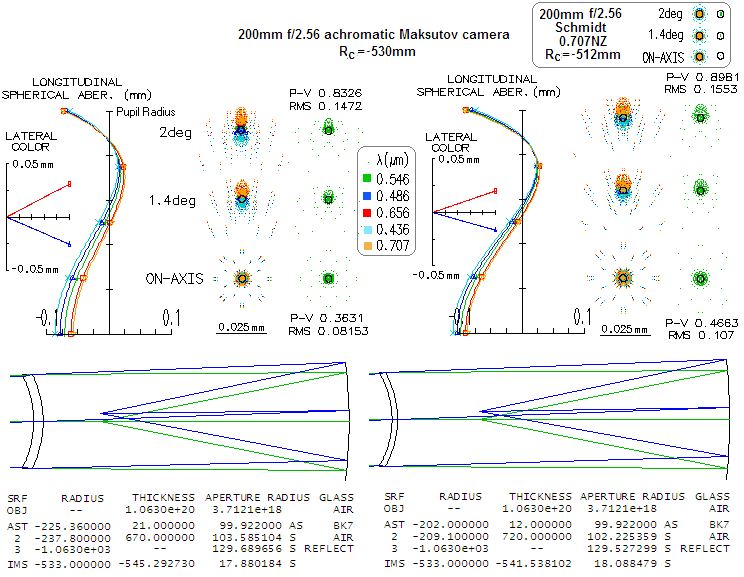FIGURE 182: Non-concentric (achromatic or Maksutov) meniscus corrector has no concentricity requirement, so the stop can be at the corrector, making the overall system more compact than the concentric meniscus, or Schmidt system. The meniscus also has no unique location; the thinner it is, the less coma it generates and the farther from mirror it has to be for corrected system coma, Also, it requires more strongly curved surfaces, in order to generate spherical aberration differential needed to offset the aberration of the mirror. As a result, Maksutov corrector generates more higher order spherical, which is making both systems less than diffraction limited in the optimized wavelength. A focal ratio closer to ƒ/3 is needed to make higher order spherical negligible. There is no appreciable longitudinal chromatism, or even spherochromatism. The variation in wavefront error with the wavelength mainly results from the wavelength variation, not the nominal wavefront error (in millimeters) itself. Most of the blur size comes from the minimized higher-order spherical aberration, which can't be eliminated in the all-spherical arrangement. The comparable Schmidt camera ray spots (top right) are noticeably smaller, especially for the optimized wavelength, the consequence of the ability of its aspheric surface to correct higher-order spherical aberration terms. The wavefront error even at the optimized e-line (546nm) is ~0.08  and 0.11 wave RMS in the Maksutov, while entirely negligible in the Schmidt. In the violet h-line (405nm), where the sphero-chromatic error in the Schmidt peaks at ~0.1 wave RMS, the mostly spherical aberration error in the Maksutov is 0.15 wave RMS with the thicker, and 0.17 wave with the thinner corrector (mostly due to more higher-order spherical in the latter). Off axis, lateral color is quite noticeable with the Maksutov, somewhat more with thinner meniscus.

While the achromatic meniscus has somewhat different properties, the system P-V wavefront error of 3rd order spherical aberration for the achromatized meniscus and a mirror still can be approximated by Eq. 120, providing the focal length of the achromatic meniscus is used. In terms of the system aberration coefficient, after substituting for the achromatic meniscus' focal length ƒL=-n2R1R2/(n-1)2t into Eq. 121, the system error given by the sum of the errors of the meniscus and the mirror, respectively, can be approximated as:As mentioned before, the peak aberration coefficient S=sD4/16 equals the P-V wavefront error at paraxial focus, or four times the error at the best (diffraction) focus. Since the ratio ƒL/R1 for non-concentric meniscus is with an ~ƒ/3 mirror roughly twice larger than for concentric meniscus, the approximation for the meniscus coefficient (first term on the right side) errs less than for concentric meniscus: it is some 3-4%, or little more, larger than the exact value for the lower-order spherical. This third-order term error is again roughly offset when the higher order term of the same sign as the third order term is included (it is also lower than with concentric meniscus, due to the greater center thickness). The inaccuracy due to the meniscus not fully behaving as thin lens is, however, larger, because of the greater center thickness of non-concentric meniscus, with the actual error being roughly 10-15% lower than what the thin lens treatment implies.

Off-axis aberrations of the Maksutov system are somewhat less complex. Being low, and of lesser importance than the axial correction, they can be described using simpler (although less accurate) approximations.

Lower-order coma of the meniscus mainly depends on its focal length and the shape factor q, which are determined by mirror properties. In effect, meniscus' coma is nearly constant at ~0.3 of mirror's coma (stop at the surface), only of opposite sign (it varies within several percent with significant changes in the meniscus' thickness).

Omitting relatively insignificant role of the position factor p and variations in the refractive index, the coma aberration coefficient of the meniscus can be approximated by cL~-1.2q/ƒL2, with q being the shape factor (R1+R2)/(R1-R2) and ƒL the meniscus focal length. The P-V wavefront error then is Wc=cαD3/12, α being the field angle. Since zero system coma requires contributions from the meniscus and the primary to offset one another, that is, the meniscus coma coefficient cL=-(1-σ)/R2, the appropriate stop (corrector) position is closely approximated by σ~1-q(R/ƒL)2. As before, σ is the corrector-to-primary separation in units of the primary radius of curvature, positive in sign.

Using the above empirical figure of the meniscus' coma as ~0.3 times the coma of the primary with the stop at the surface, the zero-coma stop position is approximated by σ~0.7, or little over 2/3 of the mirror radius of curvature. Higher-order coma of the corrector is not quite insignificant at ƒ/4 already, and grows rapidly with further increase in the focal ratio. It is also numerically negative (opposite to that of the mirror) which makes the zero-coma separation smaller: at ~ƒ/2.5 the higher-order coma of the corrector is nearly 10% of that of the mirror, resulting in the zero-coma corrector position reduced from ~0.7R (for slower systems with insignificant higher-order coma) to ~0.6R.

Obviously, for any given thickness, q will increase with the nominal increase of the radii, but the focal length will increase with the square of it, and the coma coefficient effectively decreases with the cube of the focal length increase. On the other hand, mirror coma coefficient, given by 1/R2, is inversely proportional to the square of mirror radius of curvature. From Eq. 121.1 it is evident that in balancing correction of spherical aberration the mirror radius of curvature R has to change at a faster rate than meniscus radii. This makes the rate of change in the amount of coma contributed to a system similar for both, mirror and its meniscus corrector, resulting in a relatively insignificant change of relative amounts of the aberration contributed by the two for a range of mirror focal ratios.

Meniscus' lower-order astigmatism is relatively low due to its low power. However, it is not quite negligible, and that is a positive. The aberration coefficient is approximated by aL~(n-1)t/2R1R2. Considering that the stop position is typically at σ~2/3 for the Maksutov camera, the mirror aberration coefficient of astigmatism, given by (1-σ)2/R for a sphere, is reduced by a factor of ~0.1, the two contributions - that of the mirror and that of the meniscus - are roughly similar, and opposite in sign. In other words, the stop position for cancelled system astigmatism is nearly coinciding with that needed for zero system coma.

Meniscus-type corrector is also used in various arrangements, among others in a form of single- and two-mirror catadioptric telescopes, known as Maksutov-Newtonian and Maksutov-Cassegrain, respectively.